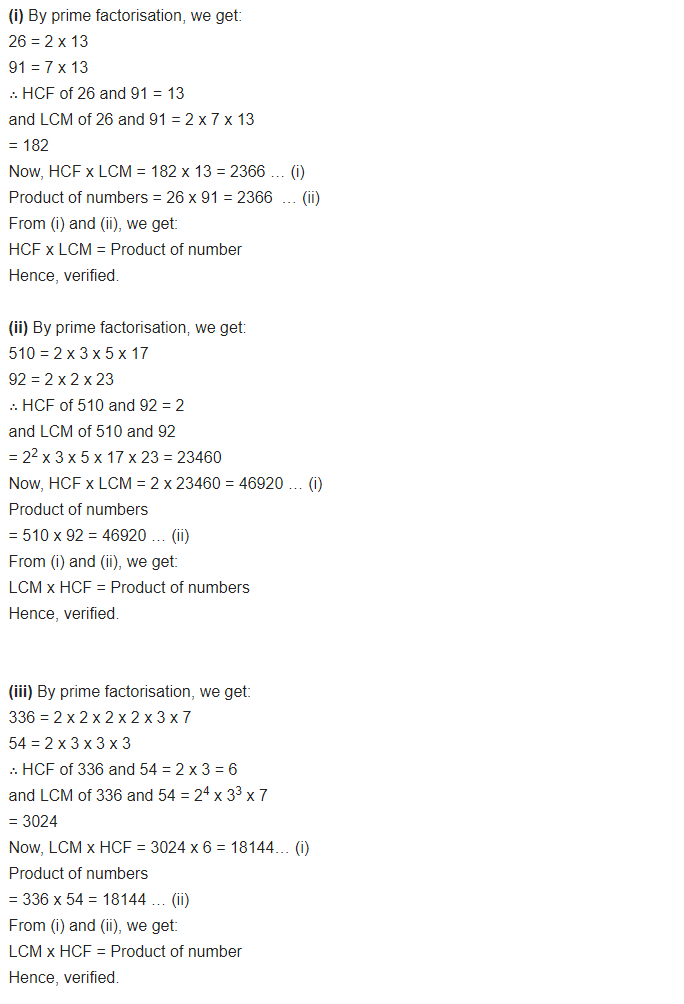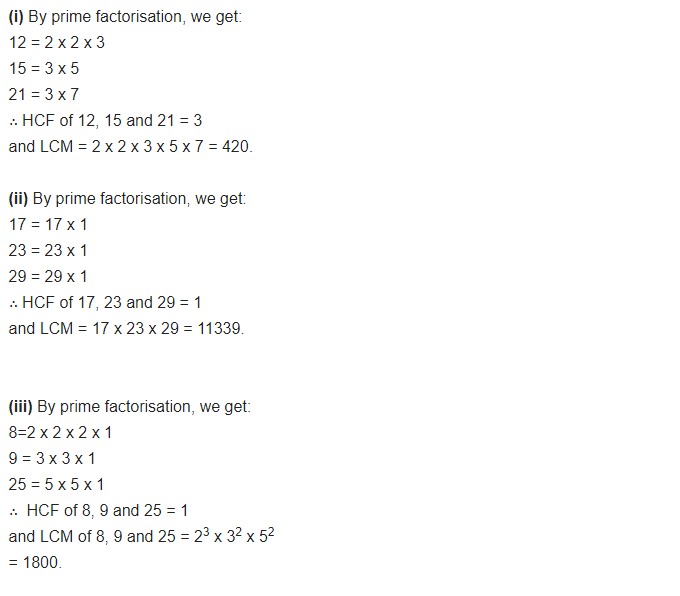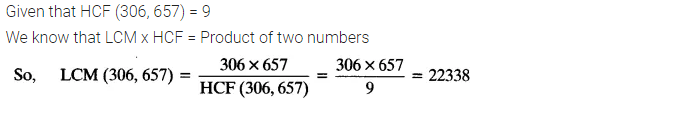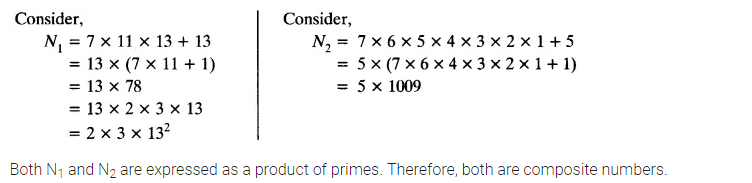ncert textbook

# NCERT Solutions for Class 10 Maths Chapter 1 Real Numbers Ex 1.2

Get Free NCERT Solutions for Class 10 Maths Chapter 1 Ex 1.2 PDF. Real Numbers Class 10 Maths NCERT Solutions are extremely helpful while doing homework. Exercise 1.2 Class 10 Maths NCERT Solutions were prepared by Experienced ncert-books.in Teachers. Detailed answers of all the questions in Chapter 1 maths class 10 Real Numbers Exercise 1.2 provided in NCERT TextBook.

Topics and Sub Topics in Class 10 Maths Chapter 1 Real Numbers:

 Section Name Topic Name 1 Real Numbers 1.1 Introduction 1.2 Euclid’s Division Lemma 1.3 The Fundamental Theorem of Arithmetic 1.4 Revisiting Irrational Numbers 1.5 Revisiting Rational Numbers and Their Decimal Expansions 1.6 Summary

You can also download the free PDF of  Ex 1.2 Class 10 Real Numbers NCERT Solutions or save the solution images and take the print out to keep it handy for your exam preparation.

 Board CBSE Textbook NCERT Class Class 10 Subject Maths Chapter Chapter 1 Chapter Name Real Numbers Exercise Ex 1.2 Number of Questions Solved 7 Category NCERT Solutions

## Maths NCERT Solutions For Class 10 Chapter 1 Ex 1.2

NCERT Solutions for Class 10 Maths Chapter 1 Real Numbers Ex 1.2 are part of NCERT Solutions for Class 10 Maths. Here we have given Maths NCERT Solutions Class 10 Chapter 1 Real Numbers Exercise 1.2

Question 1.
Express each number as a product of its prime factors:
(i) 140
(ii) 156
(iii) 3825
(iv) 5005
(v) 7429
Solution:Question 2.
Find the LCM and HCF of the following pairs of integers and verify that LCM x HCF = Product of the two numbers:
(i) 26 and 91
(ii) 510 and 92
(iii) 336 and 54
Solution:Question 3.
Find the LCM and HCF of the following integers by applying the prime factorization method:
(i) 12, 15 and 21
(ii) 17, 23 and 29
(iii) 8, 9 and 25
Solution:Question 4:
Given that HCF (306, 657) = 9, find LCM (306, 657).Question 5.
Check whether 6n can end with the digit 0 for any natural number n.Question 6.
Explain why 7 x 11 x 13 + 13 and 7 x 6 x 5 x 4 x 3 x 2 x 1 + 5 are composite numbers.Question 7.
There is a circular path around a sports field. Sonia takes 18 minutes to drive one round of the field, while Ravi takes 12 minutes for the same. Suppose they both start at the same point and at the same time and go in the same direction. After how many minutes will they meet again at the starting point?
Solution: